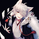Education

# Approximating A Least Square Moving Average In Pine

OANDA:EURUSD   Euro / U.S. Dollar
815 views
815
Least Squares Moving Average , Running Least Squares, Regression Line or even Running Line, this method is among the most popular ones in statistics and technical analysis .

The LSMA is extremely useful, it approximate the price pretty well and can be considered as one of the best low-lagging filters out there. Knowing how this filter is made can be really interesting. May the methods i share below inspire you to create great indicators or start coding in pine :)

A Least Squares Moving Average is defined by Tradingview as :

A line that best fits the prices specified over a user-defined time period. It is calculated using the least squares method. The result of this function is calculated using the formula: linreg = intercept + slope * (length - 1 - offset), where length is the y argument, offset is the z argument, intercept and slope are the values calculated with the least squares method on source series (x argument).

Alright, we wont use the offset parameter for our approximations, so how to calculate a least squares moving average ? If you find the mathematical formula of it you will certainly ask yourself "what are all of those maths" . But its ok, in the Pinescript you can just use the linreg() function, or you could calculate it like that :

slope = correlation(close,n,length) * (stdev(close,length)/stdev(n,length))
intercept = sma (close,length) - slope*sma(n,length)
linreg = slope*n + intercept

Ok, but can we use different estimation methods ? Certainly, the key of the LSMA is only the correlation coefficient after all, all the other parameters can be estimated.

Standard Score Or Rescaling A Line To The Price

Rescaling a line to the price is easy to do, it will give a similar result as the LSMA but it is faster to write, here the code :

A = (n - sma (n,length))/stdev(n,length) * correlation(close,n,length)
B = sma (close,length) + A*stdev(close,length)

Easier no ? We first standardized a line (n) and multiplied it by its correlation with the price, our first parameter A is dimensionless.
Then we rescaled the result to the price by multiplying our parameter with the price standard deviation and summing this result to the price moving average.

here the difference between our method and the classic LSMA of both period 100

If you put both together you wont see any difference. Overshoots can be reduced by modifying the standard deviation size.

Correlation Rescaling

The correlation coefficient is the core of a LSMA , if we rescale it we can approximate a LSMA , here the code :

a = (correlation(close,n,length) + 1)/2
b = sma (close,length) + stdev(close,length)*1.7
c = sma (close,length) - stdev(close,length)*1.7
k = c + a*(b-c)

The correlation coefficient oscillate in a range of 1/-1, we first scale it in a range of 1/0. Then you may have recognized the b and c formulas, they are the one used in bollinger bands ,
the standard deviation is multiplied by 1.7 because it was the number who best approximated a LSMA , but it could be any number defined by the user, something interesting is that this method to can fix overshoots in a classic LSMA using lower multiplier. Since our correlation is in a range of 1/0 we can rescale it to the price thanks to the method used in k.

In red our method, in blue the LSMA of both period 100.

Here the standard deviation is not multiplied by a number, this result in less overshoot.

In order to have even more manipulation over the LSMA i will try to estimate the correlation coefficient the best i can :)

So here you go, i hope you will find a use for it.

BTC : 1J5BQYnx5Wb2akoSfc3ykeShtvmXGsgP6W

ETH : 0xc418eE4039D16EDf44C52dFa292EAbdF117954B3nospamman
@nospamman, What do you mean by switching the point of correlation ? When rescaling a line to the price you don't standardize the price but only the linear function (n), this give a constant that is then multiplied by the correlation between the price and the linear function. This result is then rescaled.alexgrover
@alexgrover, i have an oscillator that goes from -1 to 1 and i tried to implement this method as an indicator. problem is, when i plot it, it plots a price relative to the line. if you swap the values for variables (n) and 'close' then i get a plot that seems to go from -1 to 1 but is correlated with price somehow. i want to plot my oscillator in relation to price, not plot the price in relation to the oscillator. does this make sense?

here's an example of what i mean:
```
a = (correlation(n, close, lsma_length) + 1)/2
b = sma (n, 5) + stdev(n, 5) * 3
c = sma (n, 5) - stdev(n, 5) * 1.7
k = c + a * (b - c)
```